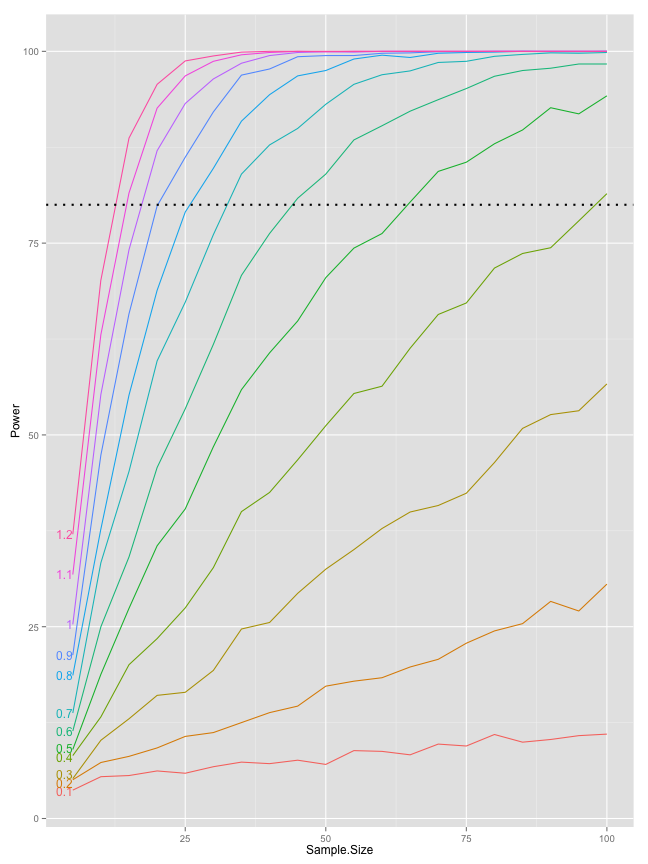## Thursday, February 13, 2014

### Continuing with Statitical Power simulation in R

Continuing with Statitical Power simulation in R

# Continuing with Statitical Power simulation in R

In the last blog post I created a simple simulation of statistical power (probability to identify effects when they are really there) calulation depending on the sample size and effect size (Cohen's D using Will Hopkins effect levels).

Now I will continue with the simulations and create 10 ES (from 0xSD [Baseline group] to 1xSD) levels with sample sizes going from 5 to 100 in 5 increment. We are going to resample 2000x

``````effect.magnitudes <- seq(from = 0, to = 1.2, length.out = 13)
subjects.list <- seq(from = 5, to = 100, by = 5)

p.value <- matrix(0, nrow = length(subjects.list), ncol = length(effect.magnitudes) -
1)

alpha <- 0.05

re.sampling <- 2000
significant.effects <- matrix(0, nrow = length(subjects.list), ncol = length(effect.magnitudes) -
1)

standard.deviation <- 30
sample.mean <- 100

for (k in 1:re.sampling) {
for (j in seq_along(subjects.list)) {
subjects <- subjects.list[j]
dataSamples <- matrix(0, nrow = subjects, ncol = length(effect.magnitudes))

for (i in seq_along(effect.magnitudes)) dataSamples[, i] <- rnorm(n = subjects,
mean = sample.mean + standard.deviation * effect.magnitudes[i],
sd = standard.deviation)

for (g in 2:(length(effect.magnitudes))) p.value[j, g - 1] <- t.test(dataSamples[,
1], dataSamples[, g])\$p.value
}

significant.effects <- significant.effects + (p.value < alpha)
}

significant.effects <- significant.effects/re.sampling * 100

significant.effects <- cbind(subjects.list, significant.effects)
colnames(significant.effects) <- c("Sample.Size", as.character(round(effect.magnitudes[-1],
2)))

significant.effects <- as.data.frame(significant.effects)
``````

We are going to plot the scores using ggplot2 and use direct labels

``````library(reshape2)
library(ggplot2)
library(directlabels)
``````
``````## Loading required package: grid
``````
``````
significant.effects.long <- melt(significant.effects, id.var = "Sample.Size",
value.name = "Power", variable.name = "Effect.Size")

gg <- ggplot(significant.effects.long, aes(x = Sample.Size, y = Power, color = Effect.Size))
gg <- gg + geom_line()
gg <- gg + geom_hline(yintercept = 80, linetype = "dotted", size = 1)
direct.label(gg, "first.qp")
``````
``````## Loading required package: proto
``````Using this graph one can estimate sample size for a wanted statistical power (usualy 80%) taking into account guessed effect size (by guessed I refer to an ES from pilot study, other research, etc). Once I get into multiple non-linear regression I can post the coefficients and create an equation. Till that happens, stay tuned :)